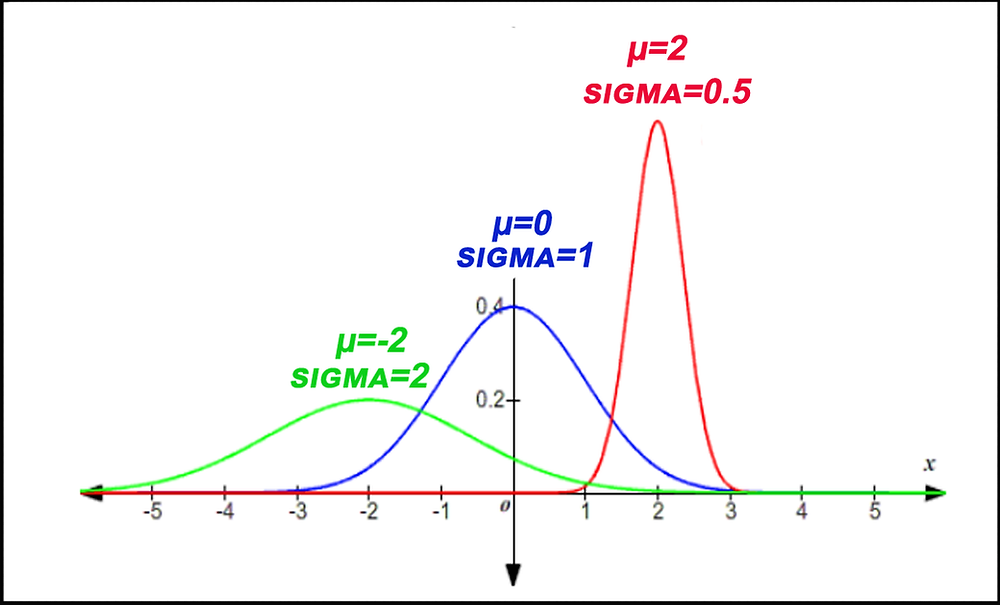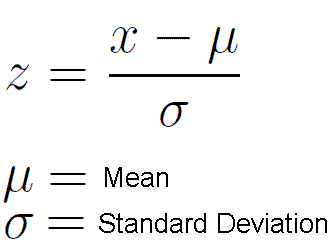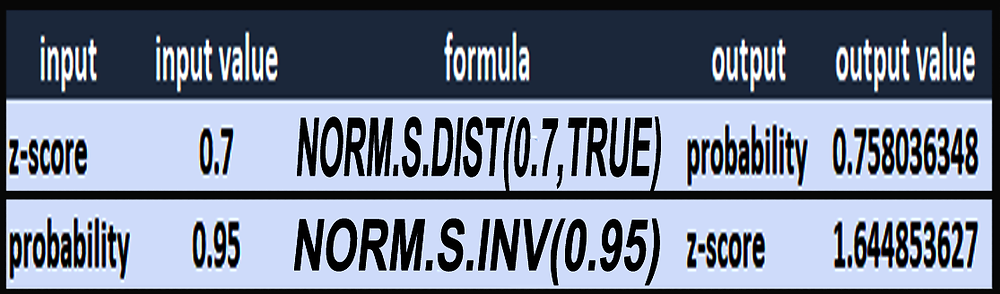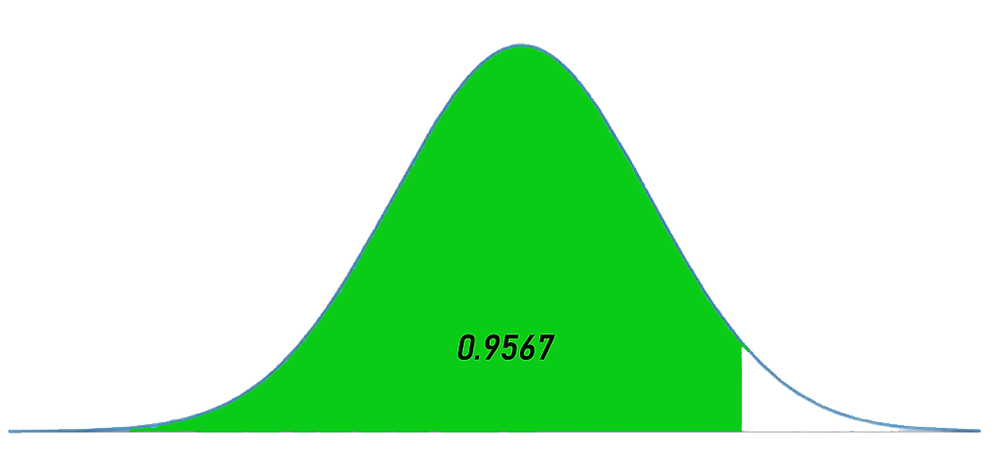Search
• Marek Vavrovic

# The Normal Distribution

Updated: May 30, 2020

Statisticians call a distribution with a bell-shaped curve a normal distribution. You may have heard of a bell curve. A bell curve describes data from a variable that has an infinite (or very large) number of possible values distributed among the population in a bell shape. This basically means a big group of individuals gravitate near the middle, with fewer and fewer individuals trailing off as you move away from the middle in either direction. Each normal distribution has its own mean, denoted by the Greek letter μ and its own standard deviation, denoted by the Greek letter σ.

The properties of any normal distribution (bell curve) are as follows:

• Always symmetrical. Asymmetrical curves display skew and are not normal.

• The distribution has a mound in the middle, with tails going down to the left and right.

• The mean is directly in the middle of the distribution. The mean does not have to be zero, and σ does not have to equal one. Area under the curve equals one.• The mean and the median are the same value because of the symmetry.

• The standard deviation is the distance from the centre to the saddle point(the place where the curve changes from an “upside-down-bowl” shape to a “right-side-up-bowl” shape.

• 68.27% of the values lie within 1 standard deviation of the mean, 95.45% lie within 2 standard deviations, and 99.73% lie within 3 standard deviations by the empirical rule.• The probability of a specific outcome is zero. We can only find the probabilities over a specific interval or range of outcomes.

Example 1

A wild group of Chihuahuas terrorizing the cats on German countryside has a mean height of 19.05 cm, with a standard deviation of 3.81cm. What proportion of these Chihuahuas are between 15.24 and 22.86 cm tall?When we want to know something about probabilities or proportions of normal distributions, we need to work with Z-scores. We use them to convert a value into the number of standard deviations it is from the mean. μ is name for the mean of the normal distribution, while σ is its standard deviation. We can find the Z-scores for 15.24 and 22.86 cm now.Excel ,z-score formulaExcel, standard deviation formulaHow much of the normal distribution falls within 1 standard deviation above or below the mean? According to the Empirical Rule, that's 68% of the distribution.

Example 2

Monthly electric bills in Wonderland are normally distributed with a mean of \$225 and a standard deviation of \$55. People in in Wonderland spend a lot of time with online gambling. In a group of 500 gamblers, how many would we expect to have a bill that is \$100 or less?

Compared to what we've worked on before, this problem only has one extra step at the end. We'll work out the proportion of the distribution that is below \$100, using the Z-score and a standard normal table, and then multiply by how many individuals we actually have.The probability of someone paying \$100 or less is 0.011521. Out of 500 people, that comes out to (0.011521)(500) = 5.76 people. We'll say "around 5 people" to keep things from getting messy.

Example 3

On a recent English test, the scores were normally distributed with a mean of 74 and a standard deviation of 7. What proportion of the class would be expected to score between 60 and 80 points?

We can't work with English scores, we need Z-scores.We need to find the probability in the middle, P(-2 <x< 0.86).

That's equal to P(x> -2) – P(x> 0.86).

The total probability under a normal curve is 1, though, so we can take 1 – P(x< -2) to find

P(x is NOT less than -2), or P(x> -2).

The normal distribution is symmetric.The upshot is that we need to find P(x > 2) and subtract it from 1. This will give us P(x > -2).P(-2 <x< 0.86) = P(x> -2) – P(x> 0.86)

= 0.97725 – 0.194894

= 0.782356

78% of the class is expected to score between 60 and 80 points on the test.

Example 4

Imagine data from Apple Corporation for 2011 – daily returns. On average, from day to day the stock went up 0.11% with standard deviation 1.84%.

What is the probability for any given day of a return greater than 0.5%?

A: We need to calculate z-score for probability 0.5%

STANDARDIZE(0.5,0.11,1.84)B: We need to calculate the probability for z-score of 0.211956522In Microsoft Excel, the following functions returns z-scores and probabilities:The first one here is the standard normal distribution function formula. What it does is, it takes the standardized z-score. You plug it into that formula and then it gives you that probability with an output value 0.758036.

Second formula stands for the inverse of the cumulative standard normal distribution. You are entering probability and getting z-score back.

Exercise 5

A company is looking to hire a new DB administrator. They give a standardized test to applicants to measure their technical knowledge. Their firs applicant, Carl, scores at 87. Based on his score, is Carl exceptionally qualified?

Based on number of previous tests, we know that the mean score is 75 out of 100, with standard deviation of 7 points.

1: convert Carl’s score to a standardized z-score using this formula:STANDARDIZE(87,75,7) = 1.714285714

2: convert z-score to probability:

NORM.S.DIST(1.71428,TRUE) = 0.9567613430.9567 represents the area to the left of Carl’s score. That percentage of people scored less than him. This means that Carl outscored 95.67% of others who took the same test. So, we know he’s basically in the top 5% of applicants.Exercise 6

Suppose that a contaminant in samples from a city’s water supply has a mean of 500 CFU/sq. cm [colony-forming units per square centimeter] and a standard deviation of 100 CFU/sq. cm. What is the probability that bacteria in a randomly selected battle of water will be:

1: LESS THAN 600 CFU/sq. cm

2: MORE THAN 600 CFU/sq. cm

3: BETWEEN 400 AND 600 CFU/sq. cm?

calculate P(x<600)=84.13%2. MORE THAN 600 CFU/sq. cm

P(x>600)= 1 - P(x<600) = 0.1584 =15.87%3: BETWEEN 400 AND 600 CFU/sq. cm

Solution #1:Solution #2:What is the probability that bacteria in a randomly selected battle of water will be between 400 AND 600 CFU/sq. cm?

P(>400<600)= 68.27%

## Recent Posts

See All

#### Index

Information functions Filter functions Table manipulation functions Grouping and summarizing Time intelligence functions OPENINGBALANCEYEAR OPENINGBALANCEYEAR(<Expression>,<Dates> [,<Filter>][,<YearEn